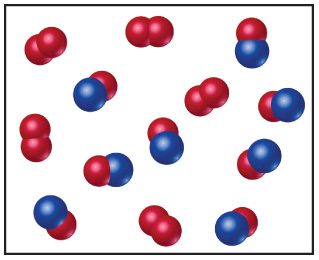# Problem: Nitrogen monoxide and oxygen react to form nitrogen dioxide. Consider the mixture of NO and O2 shown in the accompanying diagram. The blue spheres represent N, and the red ones represent O.If the actual yield of the reaction was 75% instead of 100%, how many molecules of O2 would be present after the reaction was over?

###### FREE Expert Solution

The chemical equation of the reaction is

2 NO(g) + O2(g)  2 NO2(g)

For NO,

• Given: 8 molecules NO
• Convert molecules NO to mol NO
91% (340 ratings)###### Problem Details

Nitrogen monoxide and oxygen react to form nitrogen dioxide. Consider the mixture of NO and O2 shown in the accompanying diagram. The blue spheres represent N, and the red ones represent O.If the actual yield of the reaction was 75% instead of 100%, how many molecules of O2 would be present after the reaction was over?

Frequently Asked Questions

What scientific concept do you need to know in order to solve this problem?

Our tutors have indicated that to solve this problem you will need to apply the Percent Yield concept. You can view video lessons to learn Percent Yield. Or if you need more Percent Yield practice, you can also practice Percent Yield practice problems.

What professor is this problem relevant for?

Based on our data, we think this problem is relevant for Professor Cabirac's class at ASU.